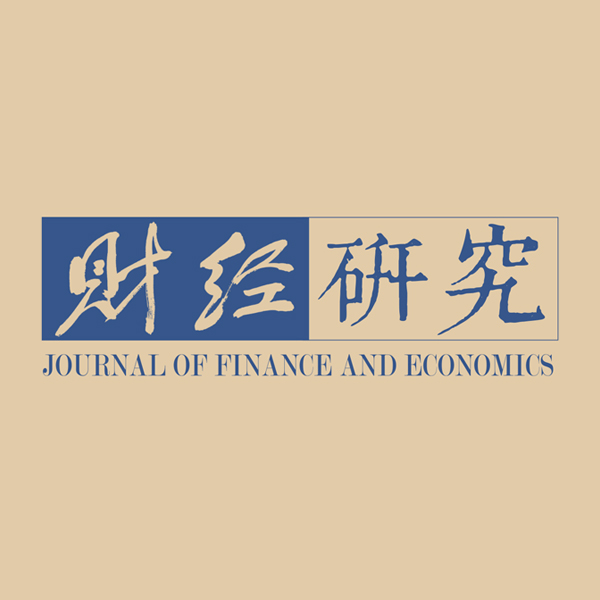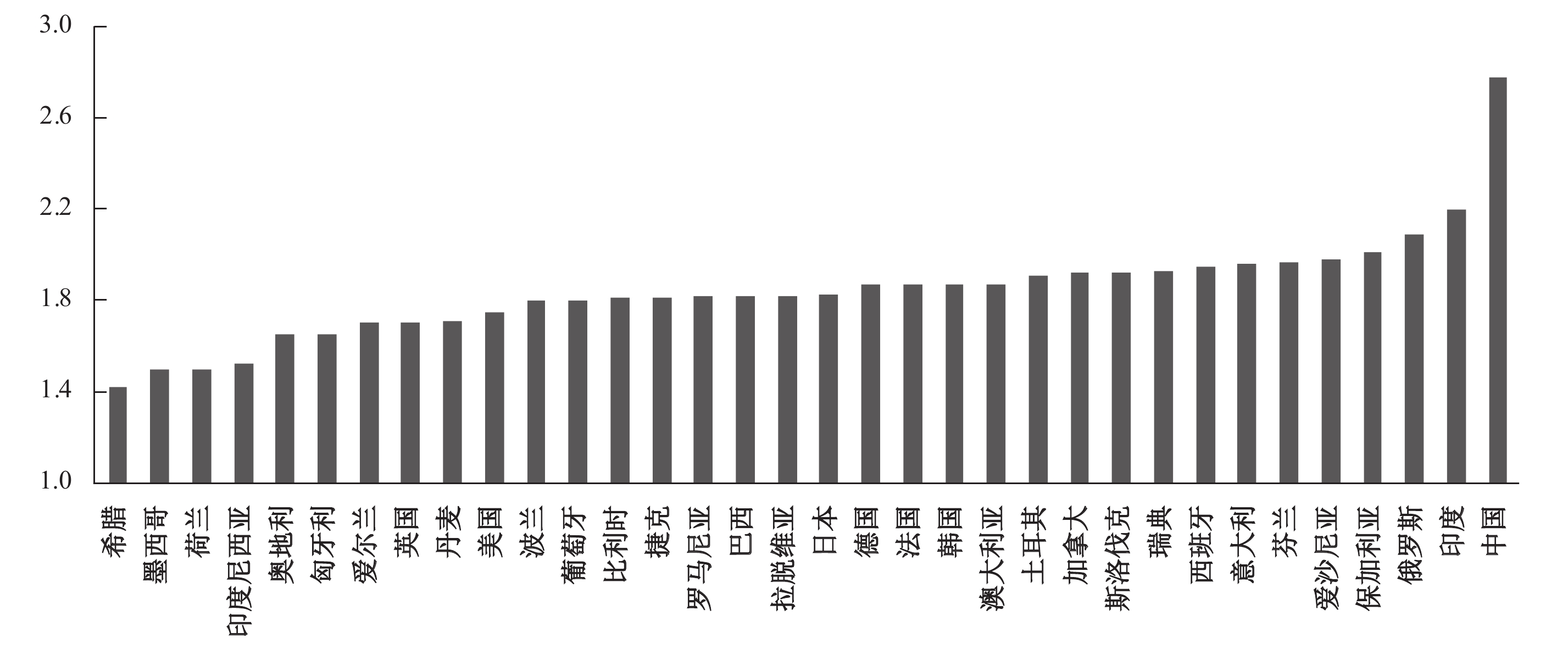﻿ 生产性服务资源嵌入制造业生产环节的最优选择《财经研究》
2020第46卷第7期

The Best Choice of Embedding Producer Service Resources in the Manufacturing Sector: From the Perspective of Upgrading the Intermediate Input Export Sophistication
Liu Hui, Chen Xiaohua, Jiang Mobing
School of Economics and Management，Zhejiang Sci-Tech University，Hangzhou 310018，China
Summary: Combing the fact of low sophistication of intermediate inputs and inefficient producer service in China with the conclusion by Zhang and Liu（2018）that producer service contributes to the improvement of intermediate production capacity and sophistication, we have the following doubt: How can producer service be embedded in the manufacturing sector to effectively promote the export sophistication of intermediate inputs? Improving the sophistication of intermediate inputs and the efficiency of producer service is fundamental to improving the quality of China’s economic growth and achieving the strategy of “address inadequacies and shore up weakness”. Therefore, it is urgent to dig into the effect of producer service resources embedded in the manufacturing sector on the intermediate input export sophistication. Our main conclusions are: When producer service resources are embedded in the manufacturing sector too upstream or downstream, they will adversely affect the intermediate input export sophistication. However, when producer service resources are embedded in the midstream of the manufacturing sector, their effect on the intermediate input export sophistication is positive and optimal. Therefore, the manufacturing section preference of producer service has an inverted U-shaped effect on the intermediate input export sophistication, and growth rate fluctuation, tax shocks and external negative shocks cannot change the inverted U-shaped mechanism. In addition, the export sophistication of China’s intermediate inputs needs to be enhanced, since the export sophistication of manufacturing and producer service intermediate inputs is lower than that of both developed and some developing countries. Our major contributions are as follows: First, we analyze the effect of producer service section preference on intermediate input export sophistication for the first time by effectively connecting export sophistication, producer service and intermediate inputs through the section preference; Second, we deepen the research in the field of export sophistication and intermediate inputs, extend the research of export sophistication from final products to intermediate products, and extend the study on intermediate products from “quantity” to “sophistication”; Third, we modify the measurement process of Antràs, et al.（2012）and Hausmann, et al.（2007）to provide two relatively reliable and scientific analysis tools for further research in this field.
Key words: producer service resources    manufacturing section    intermediate inputs    export sophistication

（一）生产性服务资源稀缺性与中间投入品出口技术复杂度

（二）生产性服务资源嵌入制造业生产环节作用机制的稳态性

（一）中间投入品出口技术复杂度的测度与分析

${X}_{ia}^{M}$
ai产业产出中被M国（AMN）作为中间投入品的金额，可测算出各国各产业中间投入品出口总额如下：

 $\left\{ \begin{array}{l} IE{X_{ia}} = X_{ia}^B + X_{ia}^C + X_{ia}^D + \cdots + X_{ia}^N \\ IE{X_{ib}} = X_{ib}^A + X_{ib}^C + X_{ib}^D + \cdots + X_{ib}^N \\ IE{X_{ic}} = X_{ic}^A + X_{ic}^B + X_{ic}^D + \cdots + X_{ic}^N \\ \mathop {}\limits_{} \mathop {}\limits_{} \mathop {}\limits_{} \mathop {}\limits_{} \mathop {}\limits_{} \mathop {}\limits_{} \mathop {}\limits_{} \mathop {}\limits_{} \mathop {}\limits_{} \mathop {}\limits_{} \vdots \\ IE{X_{in}} = X_{in}^A + X_{in}^B + X_{in}^C + \cdots + X_{in}^{N - 1} \\ \end{array} \right.$ (1)

 $PROD{Y_m} = \sum\limits_j {\frac{{IE{X_{ij}}/IE{X_j}}}{{\sum\limits_j {IE{X_{ij}}/IE{X_j}} }}{Y_j}}$ (2)

 $PRO{D_j} = \sum\limits_m {\frac{{IE{X_{mj}}}}{{\sum {IE{X_{mj}}} }}PROD{Y_m}}$ (3)

 产出投入 中间投入品 最终品 总产出 A国 B国 C国 … N国 A国 B国 C国 … N国 中间投入品 A国 ${X}_{ia}^{A}$ ${X}_{ia}^{B}$ ${X}_{ia}^{C}$ … ${X}_{ia}^{N}$ ${F}_{ia}^{A}$ ${F}_{ia}^{B}$ ${F}_{ia}^{B}$ … ${F}_{ia}^{N}$ Xia B国 ${X}_{ib}^{A}$ ${X}_{ib}^{B}$ ${X}_{ib}^{C}$ … ${X}_{ib}^{N}$ ${F}_{ib}^{A}$ ${F}_{ib}^{B}$ ${F}_{ib}^{B}$ … ${F}_{ib}^{N}$ Xib C国 ${X}_{ic}^{A}$ ${X}_{ic}^{B}$ ${X}_{ic}^{C}$ … ${X}_{ic}^{N}$ ${F}_{ic}^{A}$ ${F}_{ic}^{B}$ ${F}_{ic}^{B}$ … ${F}_{ic}^{N}$ Xic … … … … … … … … … … … … N国 ${X}_{in}^{A}$ ${X}_{in}^{B}$ ${X}_{in}^{C}$ … ${X}_{in}^{N}$ ${F}_{in}^{A}$ ${F}_{in}^{B}$ ${F}_{in}^{B}$ … ${F}_{in}^{N}$ Xin 中间投入品总投入 ${X}_{ia}^{T}$ ${X}_{ib}^{T}$ ${X}_{ic}^{T}$ … ${X}_{in}^{T}$ 增加值 ${V}_{ia}$ ${V}_{ib}$ ${V}_{ic}$ … ${V}_{in}$

（二）生产性服务资源嵌入制造业生产环节与中间投入品出口技术复杂度

 $Y_s = I_s + L_s + Z_s + F_s$ (4)

 ${Y_s} - {I_s} - {L_s} = {F_s} + {Z_s} = {F_s} + \sum\limits_{j = 1}^N {{d_{sj}}({Y_j} - {I_j} - {L_j})}$ (5)

 ${Y_s} - {I_s} - {L_s} = {F_s} + \sum\limits_{j = 1}^N {{d_{sj}}{F_j}} + \sum\limits_{j = 1}^N {\sum\limits_{k = 1}^N {{d_{sk}}{d_{kj}}{F_j} + } } \sum\limits_{j = 1}^N {\sum\limits_{k = 1}^N {\sum\limits_{l = 1}^N {{d_{sl}}{d_{lk}}{d_{kj}}{F_j}} + } } ...$ (6)

Antràs等（2012）、Antràs和Chor（2013）指出，对方程（6）右边进行加权加总可获得生产性服务资源嵌入制造业生产环节与最终产品的距离。为此，可以进一步以式（7）来刻画生产性服务资源嵌入制造业生产环节指数：

 $H{J_s} = 1 \times \frac{{{F_s}}}{{{Y_s} - {I_s} - {L_s}}} + 2 \times \frac{{\sum\limits_{j = 1}^N {{d_{sj}}{F_j}} }}{{{Y_s} - {I_s} - {L_s}}} + 3 \times \frac{{\sum\limits_{j = 1}^N {\sum\limits_{k = 1}^N {{d_{sk}}{d_{kj}}{F_j}} } }}{{{Y_s} - {I_s} - {L_s}}} + 4 \times \frac{{\sum\limits_{j = 1}^N {\sum\limits_{k = 1}^N {\sum\limits_{l = 1}^N {{d_{sl}}{d_{lk}}{d_{kj}}{F_j}} } } }}{{{Y_s} - {I_s} - {L_s}}} + \cdots$ (7)

 $H{J_s} - \sum\limits_{j = 1}^N {\frac{{{d_{sj}}({Y_j} - {I_j} - {L_j})}}{{({Y_s} - {I_s} - {L_s} - {X_s} + {M_s})}}H{J_j}} = 1$ (8)图 1 2011年34个样本国生产性服务资源嵌入制造业生产环节指数均值

 年份 下游偏好国 中游偏好国 上游偏好国 制造型 生产服务型 制造型 生产服务型 制造型 生产服务型 2000 14 224.1 15 435.5 16 219.9 16805 14 548.2 14 737.6 2002 15 247.1 16 945 17 356 18 761.5 15 507 16 441.4 2004 20 492.6 24 120.1 23 254.7 26 774.4 20 815 23 535.1 2006 23 386.5 27 722.1 26 264.8 30 540.6 23 709.8 26 970.8 2008 29 498.7 35 058.4 32 787.9 38 006.2 29 972.7 33 968.7 2010 27 130.6 31 835.3 30 200.5 34 887.1 27 531.1 30 894.9 2011 29 412.2 35 195.6 32 919 38 659.5 29 783.3 34 558.8 增幅（%） 106.78 128.02 102.96 130.05 104.72 134.49

 产业 检验类型 滞后一期 滞后两期 检验类型 滞后一期 滞后两期 制造型 Gt −3.73（0.000） −94.551（0.000） Pt −46.726（0.000） −99.080（0.000） Ga −30.1（0.000） −43.449（0.000） Pa −27.273（0.000） −47.425（0.000） 生产服务型 Gt −4.65（0.000） −37.370（0.000） Pt −61.050（0.000） −68.694（0.000） Ga −16.622（0.000） −30.086（0.000） Pa −14.413（0.000） −28.939（0.000）

（一）模型的设置与变量的选择

 $PRO{D_{jt}} = {\alpha _0} + {\alpha _1}H{J_{ijt}} + {\alpha _2}HJ_{ijt}^2 + {\gamma _m}X_{jt}^m + {\varepsilon _t}$ (9)

（二）基准模型回归结果与分析

 制造型中间投入品出口技术复杂度 生产服务型中间投入品出口技术复杂度 （1） （2） （3） （4） （1） （2） （3） （4） HJ 0.127* 0.193*** 0.210*** 0.369*** 0.192** 0.296*** 0.333*** 0.487*** （1.920） （2.905） （3.153） （5.419） （2.577） （3.948） （4.424） （6.324） HJ2 −0.206** −0.254*** −0.287*** −0.481*** −0.296*** −0.359*** −0.427*** −0.611*** （−2.420） （−3.021） （−3.367） （−5.565） （−3.068） （−3.770） （−4.439） （−6.251） YH −0.024* −0.055*** −0.056*** −0.054*** 0.008 −0.026 −0.029* −0.025 （−1.724） （−3.771） （−3.859） （−3.732） （0.529） （−1.610） （−1.775） （−1.570） DG 0.078*** 0.074*** 0.069*** 0.076*** 0.071*** 0.066*** 0.056*** 0.062*** （7.827） （7.158） （6.583） （7.079） （6.334） （5.652） （4.737） （5.148） EDU 0.160*** 0.205*** 0.206*** 0.208*** 0.203*** 0.293*** 0.296*** 0.302*** （17.42） （10.87） （10.94） （10.99） （19.60） （13.73） （13.89） （14.12） WTO 0.061*** 0.117*** 0.080*** 0.141*** （4.675） （8.268） （5.431） （8.819） RD 0.002 0.0004 0.009 −0.016 −0.019* −0.013 （0.210） （0.043） （0.921） （−1.432） （−1.740） （−1.112） FZD 0.145** 0.302*** （2.340） （4.315） GJS −0.517*** −0.494*** （−7.170） （−6.051） C 9.356*** 9.243*** 9.206*** 9.217*** 9.293*** 9.155*** 9.078*** 9.124*** （202.6） （175.0） （167.0） （176.9） （177.8） （153.3） （146.0） （154.9） Obs 3 141 2 754 2 754 2 754 3 141 2 754 2 754 2 754 R2 0.104 0.131 0.133 0.158 0.124 0.156 0.162 0.182

（三）稳健性检验结果与分析

 制造型中间投入品出口技术复杂度 生产服务型中间投入品出口技术复杂度 两步最小二乘法（2SLS） 联立方程 两步最小二乘法（2SLS） 联立方程 HJ 0.995** 2.453*** 1.760*** 2.765*** 1.480*** 2.999*** 0.431* 2.599*** （2.496） （3.532） （9.564） （12.82） （3.231） （3.754） （1.805） （11.22） HJ2 −1.515** −3.615*** −2.119*** −3.298*** −2.196*** −4.376*** −0.389* −3.091*** （−2.552） （−3.548） （−9.589） （−12.84） （−3.220） （−3.733） （−1.955） （−11.20） YH −0.022 0.008 −0.082*** −0.081*** 0.011 0.043 −0.017 −0.048** （−1.290） （0.332） （−4.905） （−4.492） （0.557） （1.629） （−0.989） （−2.514） DG 0.083*** 0.104*** 0.053*** 0.052*** 0.074*** 0.096*** 0.070*** 0.042*** （7.432） （6.720） （4.328） （3.820） （5.744） （5.422） （5.519） （2.948） EDU 0.185*** 0.216*** 0.262*** 0.289*** 0.276*** 0.312*** 0.237*** 0.364*** （8.132） （7.174） （10.80） （10.87） （10.53） （9.023） （9.287） （12.87） WTO 0.245*** 0.223*** 0.294*** 0.233*** （4.880） （11.30） （5.080） （11.12） RD 0.001 0.003 −0.019* −0.006 −0.020 −0.020 −0.002 −0.027* （0.081） （0.192） （−1.648） （−0.524） （−1.533） （−1.235） （−0.219） （−1.917） FZD 0.255** 0.282*** 0.493*** 0.248*** （2.266） （3.731） （3.810） （3.116） GJS −0.951*** −1.159*** −1.009*** −1.042*** （−4.803） （−11.06） （−4.429） （−9.351） C 9.652*** 10.19*** 9.600*** 9.805*** 9.671*** 10.290*** 8.960*** 9.680*** （61.38） （36.40） （120.6） （113.0） （53.52） （31.94） （106.3） （104.8） Obs 2 520 2 520 2 574 2 574 2 520 2 520 2 574 2 574 R2 0.249 0.302 0.065 0.272 0.286 0.347 0.088 0.064

（四）外部冲击与倒U型机制的稳固性

 M值系数 增长率 税收变化 金融冲击 制造型 生产服务型 制造型 生产服务型 制造型 生产服务型 （1） （2） （3） （4） （5） （6） HJ×M 3.415 6.252 0.034** 0.049*** 0.877*** 0.885*** （0.244） （0.401） （2.460） （3.201） （13.80） （12.37） （HJ×M）2 −2.388*** −3.041*** −0.026*** −0.035*** −0.619*** −0.588*** （−2.991） （−3.412） （−4.896） （−5.809） （−7.548） （−6.374） 控制变量 控制 控制 控制 控制 控制 控制 C 9.405*** 9.357*** 9.371*** 9.344*** 9.442*** 9.383*** （226.7） （201.9） （206.0） （184.9） （263.8） （232.9） Obs 2 862 2 862 2 628 2 628 2 862 2 862 R2 0.088 0.105 0.094 0.105 0.281 0.283

① 限于表格篇幅，本文以表1所示简表刻画WIOD2016年版投入产出表，具体表可见于WIOD网站。

② 根据WIOD投入产出表，C5-C23为制造业，C25-C56为生产性服务业，具体产业名称见WIOD2016年公布的投入产出表。在核算制造型中间投入品出口技术复杂度时，实际上是借助式（3），以各国各类制造型中间投入品产业出口额加权，即令m为制造型中间投入品产业，计算生产服务型中间投入品时，令m为各生产性服务业。限于篇幅，本文没有给出具体的计算结果；如有需要，可向作者索取。

③ 在初始阶段不考虑存货，而在测算过程中，实际上将存货归结为最终消费。当存货减少（增加）时，测度过程实际上是低估（高估）了最终消费，而本文的做法很大程度上避免了上述偏差。

④ 如果不剔除Ls，则测算出的值是该生产服务资源流向制造业上游度和流向生产性服务业上游度的加总；而剔除加总Ls后，所得结论实际上更大程度地体现了生产性服务行业s流向制造业生产环节上游度（即环节选择）。剔除流向其他生产服务业的中间品后，测度矩阵和迭代关系与原WIOD所有产业矩阵确实存在一定的差异，但由于测算所需矩阵均源于WIOD表的基础数据测算而得，因此测度结果是相对科学可靠的。感谢匿名审稿人的建议。

⑤ 线性化的具体处理方法可以参照Antràs 等（2012）一文的附件部分，进出口的处理方法也与Antràs等（2012）一文相同。

WIOD2016年公布的投入产出表将所有国家置于一张投入产出表，在数据结构上与式（8）很难匹配，因此本文以其2013年公布的数据进行测算。结合后文实证分析中控制变量的可获得性，确定后文的实证样本国为34个。WIOD2013年公布的数据中，C20-C28为生产性服务业，具体行业名称可参照WIOD2013年投入产出表。

⑦ 上游环节偏好国为2000-2011年生产性服务资源嵌入制造业环节指数均值排名前10位的国家，下游环节偏好国则为指数均值排名后10位的国家，其余14国为中游环节偏好国。限于篇幅，本文仅保留了偶数年份和2011年的测算结果；其他年份结果备索。

⑧ 本文以2012年进口排名世界前5位的国家（包括美国、中国、英国、德国和日本）为大进口国，与其中一国或一国以上相邻的国家则表示其拥有毗邻大进口国优势，

⑨ 考虑到中间投入品技术复杂度和经济发展中很多变量存在较高的相关性，为降低多重共线性可能给估计结果带来的不利影响，本文只选取税收作为第二个方程的控制变量。

⑩ 本文运用两步最小二乘法（2SLS）和联立方程对表3中两类中间品的方程（1）-方程（4）进行了稳健性检验，结果均证实了倒U形机制的存在。限于篇幅，表4仅给出了表3中方程（3）和方程（4）的稳健性检验结果。

⑪ 为了避免累赘，本文以基准方程中的方程（4）进行外部冲击检验，但前文的结果表明研发投入变量的估计结果大多不显著，因此本文在冲击检验中略去了研发投入变量。本文借用OLS、2SLS和联立方程三种方法进行了稳态性检验；限于篇幅，仅报告了2SLS的估计结果。

  陈启斐, 刘志彪. 生产性服务进口对我国制造业技术进步的实证分析[J]. 数量经济技术经济研究, 2014(3): 74–88.  陈晓华, 黄先海, 刘慧. 中国出口技术结构演进的机理与实证研究[J]. 管理世界, 2011(3): 44–57.  陈晓华, 黄先海, 刘慧. 生产性服务资源环节错配对高技术产品出口的影响分析[J]. 统计研究, 2019(1): 65–76.  陈晓华, 刘慧. 要素价格扭曲、外需疲软与中国制造业技术复杂度动态演进[J]. 财经研究, 2014(7): 119–131.  戴翔, 金碚. 产品内分工、制度质量与出口技术复杂度[J]. 经济研究, 2014(7): 4–17.  韩峰, 阳立高. 生产性服务业集聚如何影响制造业结构升级?——一个集聚经济与熊彼特内生增长理论的综合框架[J]. 管理世界, 2020(2): 72–94. DOI:10.3969/j.issn.1002-5502.2020.02.008  黄先海, 金泽成, 余林徽. 出口、创新与企业加成率: 基于要素密集度的考量[J]. 世界经济, 2018(5): 125–146.  黄先海, 宋学印. 准前沿经济体的技术进步路径及动力转换——从“追赶导向”到“竞争导向”[J]. 中国社会科学, 2017(6): 60–79.  刘海洋, 林令涛, 李倩婷. 进口中间品与中国企业生存扩延[J]. 数量经济技术经济研究, 2017(12): 58–75.  刘慧, 杨君, 吴应宇. 生产性服务资源集聚模式会影响制造业资本回报率吗[J]. 商业经济与管理, 2019(7): 75–87.  刘慧, 杨莹莹. 制造业出口技术复杂度赶超会加剧发展中国家中间品进口依赖吗[J]. 国际贸易问题, 2018(10): 31–44.  吕越, 陈帅, 盛斌. 嵌入全球价值链会导致中国制造的“低端锁定”吗?[J]. 管理世界, 2018(8): 11–29. DOI:10.3969/j.issn.1002-5502.2018.08.002  盛斌, 毛其淋. 进口贸易自由化是否影响了中国制造业出口技术复杂度[J]. 世界经济, 2017(12): 52–75.  唐晓华, 张欣珏, 李阳. 中国制造业与生产性服务业动态协调发展实证研究[J]. 经济研究, 2018(3): 79–93.  王永进, 盛丹, 施炳展, 等. 基础设施如何提升了出口技术复杂度?[J]. 经济研究, 2010(7): 103–115.  宣烨, 余泳泽. 生产性服务业集聚对制造业企业全要素生产率提升研究——来自230个城市微观企业的证据[J]. 数量经济技术经济研究, 2017(2): 89–104.  于斌斌. 生产性服务业集聚与能源效率提升[J]. 统计研究, 2018(4): 30–40.  余淼杰, 李乐融. 贸易自由化与进口中间品质量升级——来自中国海关产品层面的证据[J]. 经济学（季刊）, 2016(3): 1011–1028.  张少军, 刘志彪. 全球价值链与全球城市网络的交融——发展中国家的视角[J]. 经济学家, 2017(6): 33–41.  诸竹君, 黄先海, 余骁. 进口中间品质量、自主创新与企业出口国内增加值率[J]. 中国工业经济, 2018(8): 116–134.  Antràs P, Chor D, Fally T, et al. Measuring the upstreamness of production and trade flows[J]. American Economic Review, 2012, 102(3): 412–446. DOI:10.1257/aer.102.3.412  Antràs P, Chor D. Organizing the global value chain[J]. Econometrica, 2013, 81(6): 2127–2204. DOI:10.3982/ECTA10813  Bas M, Strauss-Kahn V. Does importing more inputs raise exports? Firm-level evidence from France[J]. Review of World Economics, 2014, 150(2): 241–275. DOI:10.1007/s10290-013-0175-0  Eswaran M, Kotwal A. The role of the service sector in the process of industrialization[J]. Journal of Development Economics, 2002, 68(2): 401–420. DOI:10.1016/S0304-3878(02)00019-6  Francois J F, Hoekman B. Services trade and policy[J]. Journal of Economic Literature, 2010, 48(3): 642–692. DOI:10.1257/jel.48.3.642  Guerrieri P, Meliciani V. Technology and international competitiveness: The interdependence between manufacturing and producer services[J]. Structural Change and Economic Dynamics, 2005, 16(4): 489–502. DOI:10.1016/j.strueco.2005.02.002  Hausmann R, Hwang J, Rodrik D. What you export matters[J]. Journal of Economic Growth, 2007, 12(1): 1–25. DOI:10.1007/s10887-006-9009-4  Johnson R C, Noguera G. Accounting for intermediates: Production sharing and trade in value added[J]. Journal of International Economics, 2012, 86(2): 224–236. DOI:10.1016/j.jinteco.2011.10.003  Krugman P R. Increasing returns, monopolistic competition, and international trade[J]. Journal of International Economics, 1979, 9(4): 469–479. DOI:10.1016/0022-1996(79)90017-5  Markusen J, Rutherford T F, Tarr D. Trade and direct investment in producer services and the domestic market for expertise[J]. Canadian Journal of Economics, 2005, 38(3): 758–777. DOI:10.1111/j.0008-4085.2005.00301.x  Schott P K. The relative sophistication of Chinese exports[J]. Economic Policy, 2008, 23(53): 6–49.  Yang F F, Yeh A G O, Wang J J. Regional effects of producer services on manufacturing productivity in China[J]. Applied Geography, 2018, 97: 263–274. DOI:10.1016/j.apgeog.2018.04.014